| my account | login-logout | resources | classroom help | support | catalog | home | get webcard |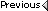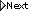» Online Classroom   »   » Public Discussion of Cel Nav   » Hawaii by Sextant

Author Topic: Hawaii by Sextant
 climber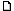posted March 19, 2016 06:52 AM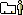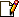I know this isn't part of rage nav course but since completing the course I have just started on the problems in Hawaii by Sextant and I don't know where to get questions answered.In the Analysis section I have been trying to understand the Automatic advancement of LOPs beginning on page 98. I cannot duplicate the a-value corrections depicted on page 99 using the equation in A1. First of all, I cannot calculate the correct value for the correction. Secondly I'm not sure whether to add or subtract the correction.In Figure A1 I am assuming that the the point at "D=S x (T2 - T1) is your DR position and that D is the distance for which you are correcting the sight. You are then advancing LOP T1 to this point using the parallel ruler. The a-value is then corrected using the distance between LOP T1 and the advanced LOP. From Figure A1 it is obvious that you would add the correction to the a-value. Is there a rule for this if you don't have it plotted? Also, the equation in Figure A1 is "a = a +D* cos(C - Zn)" while the equation in the narrative is " D * cos( Zn - C). I cannot reproduce the corrected a-values in Figure A2 using this process.Can you clarify the procedure for me? I don't know if you can answer this type of question or not - just thought I'd try.Thank you From: apalachicola
 climberposted March 19, 2016 07:34 AMI figured out part of my problem. On an iPad you have to convert degrees to radians to obtain the proper value of the Cosine of an angle - my old calculator did not require that. My bad. I can now duplicate the correction values.I still haven't found a rule for adding or subtracting the correction. Can you help there?Also, what are the axes for the plot in Figure A3? I haven't been able to figure that out although the rest of the section makes sense, so far.By the way, if I can't ask these questions here is there somewhere I can ask them?Thank you. From: apalachicola
 David Burchposted March 19, 2016 01:17 PMThis is the perfect place to ask questions about that book! From: Starpath, Seattle, WA
 climberposted March 21, 2016 11:10 AMThanks. I have a few questions.First, when advancing LOPs as in the Analysis section of the book, how do you know whether. To add or subtract the correction to the a-value?Second, what are the units for the axes in figure A3?Third, is there any way to determine which sight is the best to use in problem 3 without going through the entire sight reduction for each observation if you DON'T use a calculator? I'm trying to do as much as possible manually to be sure I understand things.Thank you. From: apalachicola
 climberposted March 21, 2016 01:11 PMA few more questions: these are for Problem 3.1. I plotted the time vs Ho points and drew a "best-fit" line through the points. I then picked what I eyeballed to be the points closest to the line. I did the sight reduction for four of the morning sights and two of the afternoon sights. I then picked the sights with the smallest a-values.I don't know if this a valid approach or not. I did not correct for distance traveled.Is this approach at all valid?2. For the :08:32:28 sight on 7 July the min. were 34.5 and the d-value was 36.8. That puts the tens at 30 and the units at 6.8. Entering the interpolation table at 34.5 for the 30's value I get 17.3, not the 19.8 in the form at the top of page 39. I get the same value for the units as the one on that form.Am I reading the table incorrectly?3. Using the 14:00:05 sight, my DR position is close to the one in box 1 of the form at the bottom of page 39. Your DR position is 41 degrees 36' N. The a-Lat used in the book is 41 degrees N. Since the minutes are 36', which is greater than 30', why wouldn't the a-Lat be 42 degrees N?Thanks for the help. From: apalachicola
 Capt Steve Millerposted March 21, 2016 01:12 PMHello Climber,I am looking into all your questions and will reply soon. From: Starpath
 climberposted March 25, 2016 01:01 PMI have one question and one comment on problem 4 in Hawaii by Sextant.1. In the worksheet on page 40, for the 13 07 23 sun sight you use an Hs of 70 degrees 38.2'. On page 13 the Hs for that sight is 70 degrees 54.0'. There is no 38.2' value. Is the 38.2' an average? Were all three sights advanced to 13 07 23? I've tried that and still can't get the 32.8' value.Can you clarify this for me?2. In the same worksheet for the 13 07 23 sight you come up with an a-Lon of 132 degrees 35.6'. However, in the plot on page 80 the AP2 is plotted as 131 degrees 35.6'. It appears that there is an error in the worksheet in box 3 where the a-lon should be entered as 131 degrees, not 132. Thank you. From: apalachicola
 David Burchposted March 25, 2016 01:35 PMCan you tell me what printing of the book you have? my copy shows 54'. Please check the copy right page, so see the date...thanksPS. if you do not have the latest we will see that you get one, as we very much appreciate your careful working of the problems. From: Starpath, Seattle, WA
 climberposted March 26, 2016 09:25 AMMy book is dated 2014. No need for me to get a new one. I used 54' and got really close to the book answer. I didn't get the book from Starpath but from another company when I ordered some other materials you don't carry.If 54' is the value to use I'm good. Thanks for clearing that up. From: apalachicola
 David Burchposted March 26, 2016 09:28 AMThere are several typos in the books that were found and fixed in the 2nd printing. Very shortly we will have a PC ebook of that printing and i can send you a s/n for that to check similar things if they might show up. we were actually planning to work on that on Monday. From: Starpath, Seattle, WA
 climberposted March 26, 2016 10:26 AMThank you.I have an overall question that has come up in problem 6 for which I have been unable to develop an understanding.Problem 6 requires one to select the best sight from 5 sights taken over a period of about 8 minutes. The first two sections of the Analysis section talk about advancing the LOPs to a common time and then plotting the calculated Hcs from the first time and the last time of the sights. Using that slope select the sight that comes closest to the line between the two calculated points.I must be dense but there seems to be a lot missing about the specifics. First of all, how do you know whether to add or subtract the corrections to the a-values(I asked this in a question above)?Secondly, how are the Hc s for the times of the first and last sights calculated? The text says a calculator is used but I would like to know if/how this is possible manually. I don't like relying on a calculator until I understand how the calculation works.I understand figures A4 and A5. What I don't understand is how to get the "Hc vs WT" line.The section on "Automatic Advancement of LOPs" doesn't seem to be tied into the next section on "Fit-Slope Method". Again, I may be dense but I can't seem to understand this.I have programmed my iPad spreadsheet to perform the calculations in Figure A3 so I understand that process. However, getting from that to Figure A5 is what I am missing. Is there any way that you can clarify this?Also, can you tell me the units for plots in Figures A3, A7, A9, etc.? It appears that these are computer-generated plots but without the x-axis units I can't tell what they represent. The bottom line is, that while I would consider purchasing a program for these calculations I don't want to do so until I can understand the process well enough to perform it manually.As far as the rest of the book, I can plot the DRs and, using the sight times in the answers section follow the navigation path very closely. However, one of the primary objects of the book is to print methods for selecting the best sight from a number of sights taken over a short period of time. This is the point I seem to be missing.Thank you. From: apalachicola
 David Burchposted March 26, 2016 11:19 AMI will address these and be right back. From: Starpath, Seattle, WA
 climberposted March 26, 2016 11:52 AMSorry to bother you with another question but I'm really having a hard time with the selection of the best sight.I've worked the sight reduction for the first and last sun sights in problem 1 to try and duplicate Figure A5. I assume that the the Hc values of 26 degrees 54.5' on the right side of the figure are typos. I get 27 degrees 04.2' for the 15 41 22 (GMT) sight and 28 degrees 27.1' for the 15 47 30 sight. These are not corrected - I used my 0844 DR position for the calculation. My DR position is within a mile of what is plotted on page 76.I'm not sure how to the corrections for the Hc calculations. I can correct the a-values using the techniques for the Automatic Advancement of LOPs but that gives you a correction to the a-value for plotting, as near as I can tell. How does that apply to the Hc correction? Do you add or subtract it from the Hc to get the corrected Hc?I'm sorry but this whole area has me confused. As I said, I can use the raw data, plot the DR plots accurately, and use the sights selected in the book to get fixes that agree with the book fixes to within 1 to 5 miles, but I do not grasp this Analysis section at all.Confused in Florida From: apalachicola
 David Burchposted March 26, 2016 12:01 PMWe will look at these points, but in the future, please limit each post to a single question or topic. it does not matter how many topics, but there are numerous reasons discussed throughout the course of the value of addressing one topic at a time. the questions you have are sort of related, but when there are so many, it takes a special circumstance to muster up the time to dive into all at once in this case, we have just had that special circumstance! Answers are marked with ======.Question 1. Problem 6 requires one to select the best sight from 5 sights taken over a period of about 8 minutes. The first two sections of the Analysis section talk about advancing the LOPs to a common time and then plotting the calculated Hcs from the first time and the last time of the sights. Using that slope select the sight that comes closest to the line between the two calculated points.==== there are two separate ways to evaluate a set of sights.(1) advance them all to a common time and then look at the list of a-values and maybe throw out any that might be way out and then average again. This is the quickest method, and likely all that is needed in most cases.(2) the second is do a slope analysis, as described in the book and which you have a question about below.we point out in the book that method (1) is not the way to get the very best analysis and in fact there are a couple examples that show that the simplest method can lead to a less than best result. More specifically, just looking at the list of advanced a values, we see one that is outside the average, but on a careful slope analysis we learn that was not the bad one, but another was the bad one.Question 2.  I must be dense but there seems to be a lot missing about the specifics. First of all, how do you know whether to add or subtract the corrections to the a-values(I asked this in a question above)?========= this is answers in Figure A-1, on page 98. you compute the new a-value according to the formula. if the result is negative, label it Away, other wise it is Toward.Question 3. Secondly, how are the Hc s for the times of the first and last sights calculated? The text says a calculator is used but I would like to know if/how this is possible manually. I don't like relying on a calculator until I understand how the calculation works.==== Calculate Hc from the times at the ends of your plot in the normal manner doing a sight reduction. These however must be done from the DR positions at the two times. This is crucial. There are ways to do sight reduction with tables from specific DR locations rather than from an assumed position but you would have to learn new tables or you would have to learn how to make special corrections to the ones done from Pub 229. (note that Pub 249 is not an option when trying to do the very best with this analysis). Thus the best way by far is do a computation of the results. there are numerous ways to do this. You can in fact have the programmed functions right in your phone. We have covered this in other places.Question 4.  I understand figures A4 and A5. What I don't understand is how to get the "Hc vs WT" line. ==== step 1 is compute the Hc values at the ends of the sight session. step 2. plot them on the same graph as your data, but you can shift the scale used as long as you keep the scale itself the same, i.e. 1 of Hc per 0.25 or whatever you choose has to be the same for data as for companions, but the center of the page vertically could be 45º 20 on one side and 45º 40 on the other side. Often the computed values will fit right on the page you have, but if not slide the scale a bit to get it on.Then to get the other lines, you are just using parallel rulers or rolling plotter to move that line up and down the page to look for the best fit.Question 5. The section on "Automatic Advancement of LOPs" doesn't seem to be tied into the next section on "Fit-Slope Method". Again, I may be dense but I can't seem to understand this.==== it is not tied in as it is not related. as noted above, these are two different analysis methods.Question 6. I have programmed my iPad spreadsheet to perform the calculations in Figure A3 so I understand that process. However, getting from that to Figure A5 is what I am missing. Is there any way that you can clarify this?==== again, we mix up two methods here. Figure A3 is a plot of the sights all advanced to the same time and then all plotted. No averaging of the a-values. This is a way to visually see how well the sights agree with each other. In this case we have two sets of sights. One set (s) had 4 sights, and the other set (v) had 3 sights. And we see from that plot that the data are not very good (the logbook even warned of that) so we look to a new method of analysis, i.e. we start the fit slope analysis for the 4 sun sighs and that is plotted in A5. So A3 just showed that we needed bigger guns to try to get better results, so we switch gears and do the analysis of A5.Question 7.Also, can you tell me the units for plots in Figures A3, A7, A9, etc.? It appears that these are computer-generated plots but without the x-axis units I can't tell what they represent. === the scales of these plots are shown in big numbers right on the drawing, so i do not follow this one. In other words, use a ruler to measure the distance marked at 33 long (in my book i get 81mm, so the scale of that one is 33 to 81mm.Question 8.  The bottom line is, that while I would consider purchasing a program for these calculations I don't want to do so until I can understand the process well enough to perform it manually.====== you do not need to purchase anything to get these computations, there are numerous free apps for iOS and Android and for PC and for MacQuestion 9. As far as the rest of the book, I can plot the DRs and, using the sight times in the answers section follow the navigation path very closely. However, one of the primary objects of the book is to print methods for selecting the best sight from a number of sights taken over a short period of time. This is the point I seem to be missing.===== i hope the above notes helps with this. From: Starpath, Seattle, WA
 David Burchposted March 26, 2016 12:10 PMI see what may be a stumbling block. The original topic title Hawaii by Sextant is too general. Somehow we missed that. Future topics should be please very specific, ie Hawaii by Sextant, sight analysis, page xx or section xx, and so on. That will help us keep organized here and make it easier for others to look up what they might want to find.I think the notes above might help with the most recent post. So we will let that rest for a while. From: Starpath, Seattle, WA

 All times are Pacific